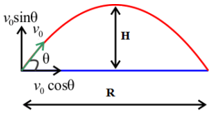# Projectile Motion Formula

## Projectile Motion Formulas - Concepts and Solved Examples

There are many types of motion around us. Projectile motion is a form of motion experienced by an object or particle that is thrown near the Earth's surface and moves along a curved path under the action of gravity only (in particular, the effects of air resistance are assumed to be negligible). Such an object is called a projectile and the curved path with which the projectile travels is what is termed as trajectory.

Formulae related to a projectile that is launched at an oblique angle with respect to the horizontal and whose motion is referred with respect to the horizontal are:(v0 is the velocity of projection, θ

Question: If the horizontal range of a projectile is 4 times the maximum height attained by it, then the angle of projection is:
Options:
(a) 45°
(b) 30°
(c) 60°
(d) 15°